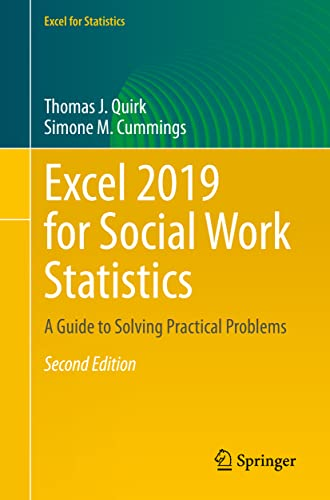# Excel 2019 for Social Work Statistics, 2nd Edition

Rating: 0 out of 5 0 reviews
• Length: 281 pages
• Edition: 2
• Publisher:
• Publication Date: 2021-04-02
• ISBN-10: 3030682560
• ISBN-13: 9783030682569

## Book Description

This text is a step-by-step, exercise-driven guide for students and practitioners who need to master Excel to solve practical social work problems. If understanding statistics isn’t your strongest suit, you are not especially mathematically inclined, or if you are wary of computers, this is the right book for you.

Excel, a widely available computer program for students and managers, is also an effective teaching and learning tool for quantitative analyses in social work courses. Its powerful computational ability and graphical functions make learning statistics much easier than in years past. Excel 2019 for Social Work Statistics: A Guide to Solving Practical Problems capitalizes on these improvements by teaching students and managers how to apply Excel to statistical techniques necessary in their courses and work.

In this new edition, each chapter explains statistical formulas and directs the reader to use Excel commands to solve specific, easy-to-understand social work problems. Practice problems are provided at the end of each chapter with their solutions in an Appendix. Separately, there is a full Practice Test (with answers in an Appendix) that allows readers to test what they have learned.

``````1. Sample Size, Mean, Standard Deviation, and Standard Error of the Mean
2. Random Number Generator
3. Confidence Interval About the Mean Using the TINV Function and Hypothesis Testing
4. One-Group t-Test for the Mean
5. Two-Group t-Test of the Difference of the Means for Independent Groups
6. Correlation and Simple Linear Regression
7. Multiple Correlation and Multiple Regression
8. One-Way Analysis of Variance (ANOVA)``````